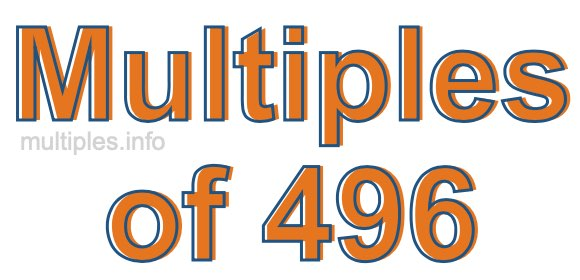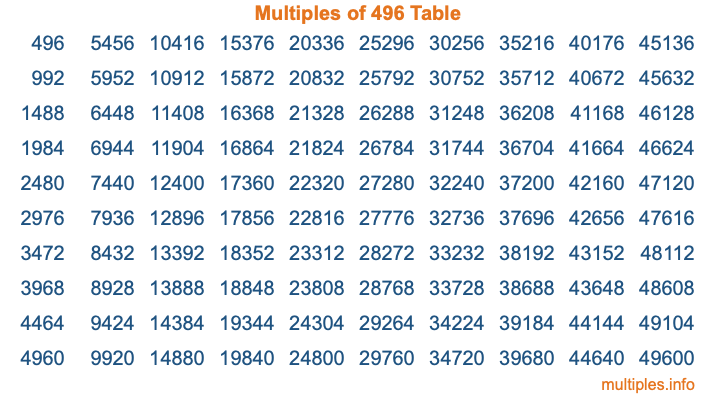Multiples of 496Welcome to the Multiples of 496 page. Here we will first teach you everything you will ever need to know about the multiples of 496, and then give you a study guide summary of everything we taught you to make sure you remember it all. Use this page to look up facts and learn information about the multiples of 496. This page will make you a multiples of four hundred ninety-six expert!

Definition of Multiples of 496
Multiples of 496 are all the numbers that when divided by 496 equal an integer. Each of the multiples of 496 are called a multiple. A multiple of 496 is created by multiplying 496 by an integer.

Therefore, to create a list of multiples of 496, you start with 1 multiplied by 496, then 2 multiplied by 496, then 3 multiplied by 496, and so on for as long as you want. Thus, the list of the first five multiples of 496 is 496, 992, 1488, 1984, and 2480. To see a larger list of multiples of 496, see the printable image of Multiples of 496 further down on this page. We also have a category where you can choose any nth multiple of 496.

Multiples of 496 Checker
The Multiples of 496 Checker below checks to see if any number of your choice is a multiple of 496. In other words, it checks to see if there is any number (integer) that when multiplied by 496 will equal your number. To do that, we divide your number by 496. If the the quotient is an integer, then your number is a multiple of 496.

Is  a multiple of 496?

Least Common Multiple of 496 and ...
A Least Common Multiple (LCM) is the lowest multiple that two or more numbers have in common. This is also called the smallest common multiple or lowest common multiple and is useful to know when you are adding our subtracting fractions. Enter one or more numbers below (496 is already entered) to find the LCM.

Check out our LCM Calculator if you need more details about the Least Common Multiple or if you need the LCM for different numbers for adding and subtraction fractions.

nth Multiple of 496
As we stated above, 496 is the first multiple of 496, 992 is the second multiple of 496, 1488 is the third multiple of 496, and so on. Enter a number below to find the nth multiple of 496.

th multiple of 496

Multiples of 496 vs Factors of 496
496 is a multiple of 496 and a factor of 496, but that is where the similarities end. All postive multiples of 496 are 496 or greater than 496. All positive factors of 496 are 496 or less than 496.

Below is the beginning list of multiples of 496 and the factors of 496 so you can compare:

Multiples of 496: 496, 992, 1488, 1984, 2480, etc.

Factors of 496: 1, 2, 4, 8, 16, 31, 62, 124, 248, 496

As you can see, the multiples of 496 are all the numbers that you can divide by 496 to get a whole number. The factors of 496, on the other hand, are all the whole numbers that you can multiply by another whole number to get 496.

It's also interesting to note that if a number (x) is a factor of 496, then 496 will also be a multiple of that number (x).

Multiples of 496 vs Divisors of 496
The divisors of 496 are all the integers that 496 can be divided by evenly. Below is a list of the divisors of 496.

Divisors of 496: 1, 2, 4, 8, 16, 31, 62, 124, 248, 496

The interesting thing to note here is that if you take any multiple of 496 and divide it by a divisor of 496, you will see that the quotient is an integer.

Multiples of 496 Table
Below is an image of the first 100 multiples of 496 in a table. The table is in chronological order, column by column. The first column has the first ten multiples of 496, the second column has the next ten multiples of 496, and so on.The Multiples of 496 Table is also referred to as the 496 Times Table or Times Table of 496. You are welcome to print out our table for your studies.

Negative Multiples of 496
Although not often discussed or needed in math, it is worth mentioning that you can make a list of negative multiples of 496 by multiplying 496 by -1, then by -2, then by -3, and so on, to get the following list of negative multiples of 496:

-496, -992, -1488, -1984, -2480, etc.

Multiples of 496 Summary
Below is a summary of important Multiples of 496 facts that we have discussed on this page. To retain the knowledge on this page, we recommend that you read through the summary and explain to yourself or a study partner why they hold true.

There are an infinite number of multiples of 496.

A multiple of 496 divided by 496 will equal a whole number.

496 divided by a factor of 496 equals a divisor of 496.

The nth multiple of 496 is n times 496.

The largest factor of 496 is equal to the first positive multiple of 496.

496 is a multiple of every factor of 496.

496 is a multiple of 496.

A multiple of 496 divided by a divisor of 496 equals an integer.

496 divided by a divisor of 496 equals a factor of 496.

Any integer times 496 will equal a multiple of 496.

Multiples of a Number
Here you can get the multiples of another number, all with the same attention to detail as we did for multiples of 496 on this page.

Multiples of
Multiples of 497
Did you find our page about multiples of four hundred ninety-six educational? Do you want more knowledge? Check out the multiples of the next number on our list!